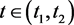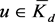﻿ 一类非局部边值问题正解的存在性

# 一类非局部边值问题正解的存在性The Existence of Positive Solutions for a Nonlocal Boundary Value ProblemAbstract: This paper discusses the boundary value problem with nonlocal integral boundary conditions

Abstract:Abstract: where,; A and B are functions of bounded variation;,; the nonlinearityis continuous and is allowed to change sign. According to the fixed point theorem in double cones, we obtain that there exists at least two positive solutions. And according to three-solution theorem, we obtain that there exists at least three positive solutions.

Abstract:

1. 引言

$\left\{\begin{array}{l}{u}^{″}\left(t\right)+q\left(t\right)f\left(t,u\left(t\right)\right)=0,0 (1.1)

$\alpha \left[u\right]={\int }_{0}^{1}u\left(t\right)\text{d}A\left(t\right),\beta \left[u\right]={\int }_{0}^{1}u\left(t\right)\text{d}B\left(t\right).$

2000年，Guidotti和Merino在文献  中研究了

$\left\{\begin{array}{l}-{u}^{″}\left(t\right)=y\left(t\right),t\in \left(0,1\right),\hfill \\ {u}^{\prime }\left(0\right)=0,{u}^{\prime }\left(1\right)+\beta u\left(0\right)=0\left(\beta >0\right).\hfill \end{array}$

2006年，Webb和Infante在文献  中研究了以下方程正解的存在性问题：

$\left\{\begin{array}{l}-{u}^{″}\left(t\right)=g\left(t\right)f\left(t,u\right),0

2012年，Webb在文献  中研究了温控器模型正解的存在性：

$\left\{\begin{array}{l}-{u}^{″}\left(t\right)=g\left(t\right)f\left(t,u\right),0

2015年，Infante在文献  中研究了Neumann型非局部边值问题

$\left\{\begin{array}{l}{u}^{″}\left(t\right)+f\left(t,u\left(t\right)\right)=0,t\in \left[0,1\right],\hfill \\ {u}^{\prime }\left(0\right)=\alpha \left[u\right],\hfill \\ {u}^{\prime }\left(1\right)=\beta \left[u\right].\hfill \end{array}$

$\left(Tu\right)\left(t\right)=\gamma \left(t\right)\alpha \left[u\right]+\delta \left(t\right)\beta \left[u\right]+{\int }_{0}^{1}k\left(t,s\right)g\left(s\right)f\left(s,u\left(s\right)\right)\text{d}s,$

2. 预备知识和引理

(H1) $a>0,b>0$

(H2) $f\in C\left(\left[0,1\right]×\left[0,\infty \right),R\right),f\left(t,0\right)>0,\forall t\in \left[0,1\right]$

(H3) $A\left(t\right),B\left(t\right)$ 是有界变差函数，且 ${\int }_{0}^{1}\text{d}A\left(t\right)\triangleq \alpha \left[\stackrel{^}{1}\right]>0,{\int }_{0}^{1}\text{d}B\left(t\right)\triangleq \beta \left[\stackrel{^}{1}\right]>0$

$\left\{\begin{array}{l}{u}^{″}\left(t\right)=0,t\in \left(0,1\right),\hfill \\ au\left(0\right)-b{u}^{\prime }\left(0\right)=0,\hfill \\ {u}^{\prime }\left(1\right)=0.\hfill \end{array}$

$G\left(t,s\right)=\left\{\begin{array}{l}\frac{1}{a}\left(as+b\right),s\le t,\hfill \\ \frac{1}{a}\left(at+b\right),t\le s.\hfill \end{array}$

${G}_{t}\left(t,s\right)=\left\{\begin{array}{l}0,s\le t,\hfill \\ 1,t\le s,\hfill \end{array}$

$\frac{b}{a+b}G\left(s,s\right)\le G\left(t,s\right)\le G\left(s,s\right),\forall t,s\in \left[0,1\right].$

$X=C\left[0,1\right]=\left\{u:u为\left[0,1\right]上的连续函数\right\}$ 。对 $u\in X$ ，定义

$‖u‖=\underset{t\in \left[0,1\right]}{\mathrm{max}}|u\left(t\right)|,$

$\left(X,‖\cdot ‖\right)$ 为一Banach空间。 $K=\left\{\mathrm{u\in X}:\mathrm{u \left(t \right)}\ge 0\right\}$

${K}^{\prime }=\left\{u\in X:u\left(t\right)\ge \frac{b}{a+b}‖u‖\right\},$

$\theta \left(u\right)=\underset{t\in \left[0,1\right]}{\mathrm{min}}|u\left(t\right)|.$

${\left(\cdot \right)}^{+}=\mathrm{max}\left\{\cdot ,0\right\}$ ，我们定义算子 $T,A,{T}^{*}$ 如下：

$T:K\to K,A:K\to X,{T}^{*}:{K}^{\prime }\to {K}^{\prime },$

$\left(Tu\right)\left(t\right)={\left[\frac{1}{a}\alpha \left[u\right]+\left(\frac{b}{a}+t\right)\beta \left[u\right]+{\int }_{0}^{1}G\left(t,s\right)q\left(s\right)f\left(s,u\left(s\right)\right)\text{d}s\right]}^{+},t\in \left[0,1\right],$

$\left({T}^{*}u\right)\left(t\right)=\frac{1}{a}\alpha \left[u\right]+\left(\frac{b}{a}+t\right)\beta \left[u\right]+{\int }_{0}^{1}G\left(t,s\right)q\left(s\right){f}^{+}\left(s,u\left(s\right)\right)\text{d}s,t\in \left[0,1\right].$

$\forall u\in {K}^{\prime }$ ，有 ${T}^{*}u\in C\left[0,1\right]$ ，且

$\begin{array}{c}\left({T}^{*}u\right)\left(t\right)=\frac{1}{a}\alpha \left[u\right]+\left(\frac{b}{a}+t\right)\beta \left[u\right]+{\int }_{0}^{1}G\left(t,s\right)q\left(s\right){f}^{+}\left(s,u\left(s\right)\right)\text{d}s\\ \ge \frac{1}{a}\alpha \left[u\right]+\left(\frac{b}{a}+t\right)\beta \left[u\right]+\frac{b}{a+b}{\int }_{0}^{1}G\left(s,s\right)q\left(s\right){f}^{+}\left(s,u\left(s\right)\right)\text{d}s\\ \ge \frac{b}{a+b}\left(\frac{1}{a}\alpha \left[u\right]+\left(\frac{b}{a}+t\right)\beta \left[u\right]+\frac{b}{a+b}{\int }_{0}^{1}G\left(s,s\right)q\left(s\right){f}^{+}\left(s,u\left(s\right)\right)\text{d}s\right)\\ \ge \frac{b}{a+b}‖{T}^{*}u‖.\end{array}$

$\left(Au\right)\left(t\right)\ge 0,\forall t\in \left[0,1\right].$

${\left(Au\right)}^{\prime \text{​}\prime }\left(t\right)=-q\left(t\right)f\left(t,0\right)<0,t\in \left({t}_{1},{t}_{2}\right).$

1) ${t}_{1}=0$

${t}_{2}<1$ ，则 $\left(Au\right)\left({t}_{2}\right)=0$ ，且 $\left(Au\right)\left(t\right)<0,t\in \left({t}_{1},{t}_{2}\right)$ ，而时， ${\left(Au\right)}^{\prime \text{​}\prime }\left(t\right)<0$ ，即 $Au$$\left[{t}_{1},{t}_{2}\right]$ 上为凹函数，所以 ${\left(Au\right)}^{\prime }\left(t\right)>0$ 。但又

$\alpha \left(Au\right)\left(0\right)-b{\left(Au\right)}^{\prime }\left(0\right)=\alpha \left[u\right],$

$\left(Au\right)\left(0\right)=\frac{b{\left(Au\right)}^{\prime }\left(0\right)+\alpha \left[u\right]}{b}>0.$

${t}_{2}=1$ ，此时，且，故

$\left(Au\right)\left(t\right)=\frac{1}{a}\alpha \left[0\right]+\left(\frac{b}{a}+t\right)\beta \left[0\right]+{\int }_{0}^{1}G\left(t,s\right)q\left(s\right)f\left(s,0\right)\text{d}s,t\in \left[0,1\right].$

2) ${t}_{1}>0$

${t}_{2}<1$ ，则 $\left(Au\right)\left({t}_{1}\right)=0,\left(Au\right)\left({t}_{2}\right)=0$ ，且 $\left(Au\right)\left(t\right)<0,t\in \left({t}_{1},{t}_{2}\right)$ 于是${\left(Au\right)}^{\prime \text{​}\prime }\left(t\right)=-q\left(t\right)f\left(t,0\right)<0,t\in \left({t}_{1},{t}_{2}\right)$

$\left(Au\right)\left(t\right)>\left(Au\right)\left({t}_{1}\right)=\left(Au\right)\left({t}_{2}\right)=0.$

${t}_{2}=1$ ，则 $\left(Au\right)\left({t}_{1}\right)=0,\left(Au\right)\left(t\right)<0,t\in \left({t}_{1},{t}_{2}\right)$ ，于是

$u\left(t\right)=0,t\in \left[{t}_{1},{t}_{2}\right],\text{\hspace{0.17em}}{\left(Au\right)}^{\prime \text{​}\prime }\left(t\right)=-q\left(t\right)f\left(t,0\right)<0,t\in \left({t}_{1},{t}_{2}\right).$

(C1) 当 $\left\{u\in P\left(a,b,d\right):\alpha \left(u\right)>b\right\}\ne \varnothing$ ，且 $u\in P\left(a,b,d\right)$ 时，恒有 $\alpha \left(Au\right)>b$

(C2) 当 $u\in {\stackrel{¯}{P}}_{a}$ 时，恒有 $‖Au‖

(C3) 当 $u\in P\left(a,b,c\right)$$‖Au‖>d$ 时，恒有 $\alpha \left(Au\right)>b$

(M1) $‖Tx‖

(M2) $‖{T}^{*}x‖ ，且 $\theta \left({T}^{*}x\right)>b,x\in \partial {K}^{\prime }\left(b\right)$

(M3) $Tx={T}^{*}x,x\in {{K}^{\prime }}_{a}\left(b\right)\cap \left\{x:{T}^{*}x=x\right\}$

$0\le ‖{x}_{1}‖

3. 主要结果

(H4) $f\left(t,u\right)\ge 0,u\in \left[d,R\right]$

(H5) $|f\left(t,u\right)|\le Mr,\left(t,u\right)\in \left[0,1\right]×\left[0,r\right]$

(H6) $|f\left(t,u\right)|\ge mr,\left(t,u\right)\in \left[0,1\right]×\left[\frac{b}{a+b}\mathrm{R.R}\right]$

(H7) $\frac{\alpha \left[\stackrel{^}{1}\right]}{a}+\left(1+\frac{b}{a}\right)\beta \left[\stackrel{^}{1}\right]+M{\int }_{0}^{1}G\left(s,s\right)q\left(s\right)\text{d}s<1$

(H8) $\frac{b}{a+b}\left(\frac{\alpha \left[\stackrel{^}{1}\right]}{a}+\frac{b}{a}\beta \left[\stackrel{^}{1}\right]+m{\int }_{0}^{1}G\left(s,s\right)q\left(s\right)\text{d}s\right)>1$

(H9) $d<\frac{b}{a+b}R$

$0<‖{u}_{1}‖

$‖Tu‖=\underset{t\in \left[0,1\right]}{\mathrm{max}}{\left[\frac{1}{a}\alpha \left[u\right]+\left(\frac{b}{a}+t\right)\beta \left[u\right]+{\int }_{0}^{1}G\left(t,s\right)q\left(s\right)f\left(s,u\left(s\right)\right)\text{d}s\right]}^{+},$

$\begin{array}{c}‖Tu‖=\underset{t\in \left[0,1\right]}{\mathrm{max}}\mathrm{max}\left\{\frac{1}{a}\alpha \left[u\right]+\left(\frac{b}{a}+t\right)\beta \left[u\right]+{\int }_{0}^{1}G\left(t,s\right)q\left(s\right){f}^{+}\left(s,u\left(s\right)\right)\text{d}s,0\right\}\\ \le \frac{\alpha \left[\stackrel{^}{1}\right]}{a}r+\left(1+\frac{b}{a}\right)\beta \left[\stackrel{^}{1}\right]r+Mr{\int }_{0}^{1}G\left(s,s\right)q\left(s\right)\text{d}s\\ =\left(\frac{\alpha \left[\stackrel{^}{1}\right]}{a}+\left(1+\frac{b}{a}\right)\beta \left[\stackrel{^}{1}\right]+M{\int }_{0}^{1}G\left(s,s\right)q\left(s\right)\text{d}s\right)r\\

$\begin{array}{c}‖{T}^{*}u‖=\underset{t\in \left[0,1\right]}{\mathrm{max}}\left\{\frac{1}{a}\alpha \left[u\right]+\left(\frac{b}{a}+t\right)\beta \left[u\right]+{\int }_{0}^{1}G\left(t,s\right)q\left(s\right){f}^{+}\left(s,u\left(s\right)\right)\text{d}s,0\right\}\\ \le \frac{\alpha \left[\stackrel{^}{1}\right]}{a}r+\left(1+\frac{b}{a}\right)\beta \left[\stackrel{^}{1}\right]r+Mr{\int }_{0}^{1}G\left(s,s\right)q\left(s\right)\text{d}s\\

$u\in \partial {K}^{\prime }\left(\frac{b}{a+b}R\right)$ ，即 $u\in {K}^{\prime }$ ，且 $\theta \left(u\right)=\underset{t\in \left[0,1\right]}{\mathrm{min}}u\left(t\right)=\frac{b}{a+b}R$ ，我们有

$\frac{b}{a+b}R=\theta \left(u\right)=\underset{t\in \left[0,1\right]}{\mathrm{min}}u\left(t\right)\ge \frac{b}{a+b}‖u‖,$

$‖u‖\le R.$

$u\left(t\right)\ge \frac{b}{a+b}R,\forall t\in \left[0,1\right],$

$\frac{b}{a+b}R\le u\left(t\right)\le ‖u‖\le R,\forall t\in \left[0,1\right].$

$\begin{array}{c}\left({T}^{*}u\right)\left(t\right)=\frac{1}{a}\alpha \left[u\right]+\left(\frac{b}{a}+t\right)\beta \left[u\right]+{\int }_{0}^{1}G\left(t,s\right)q\left(s\right){f}^{+}\left(s,u\left(s\right)\right)\text{d}s\\ \ge \frac{\alpha \left[\stackrel{^}{1}\right]}{a}\frac{b}{a+b}R+\frac{b}{a}\beta \left[\stackrel{^}{1}\right]\frac{b}{a+b}R+mR{\int }_{0}^{1}\frac{b}{a+b}G\left(s,s\right)q\left(s\right)\text{d}s\\ =\frac{b}{a+b}\left(\frac{\alpha \left[\stackrel{^}{1}\right]}{a}+\left(1+\frac{b}{a}\right)\beta \left[\stackrel{^}{1}\right]+m{\int }_{0}^{1}G\left(s,s\right)q\left(s\right)\text{d}s\right)R\\ >R.\end{array}$

$\theta \left({T}^{*}u\right)=\underset{t\in \left[0,1\right]}{\mathrm{min}}\left({T}^{*}u\right)\left(t\right)\ge \frac{b}{a+b}‖{T}^{*}u‖>\frac{b}{a+b}R,$

$\theta \left({T}^{*}u\right)>\frac{b}{a+b}R.$

$u\in \partial {{K}^{\prime }}_{r}\left(\frac{b}{a+b}R\right)\cap \left\{u:{T}^{*}u=u\right\}$ ，即 $u\in {K}^{\prime }$ ，且 $‖u‖>r,\theta \left(u\right)=\underset{t\in \left[0,1\right]}{\mathrm{min}}u\left(t\right)<\frac{b}{a+b}R$

$u\left(t\right)\ge \frac{b}{a+b}‖u‖,$

$\frac{b}{a+b}‖u‖\le \underset{t\in \left[0,1\right]}{\mathrm{min}}u\left(t\right)<\frac{b}{a+b}R,$

$‖u‖

$\frac{b}{a+b}r\le \frac{b}{a+b}‖u‖\le u\left(t\right)\le ‖u‖

$d

${f}^{+}\left(t,u\left(t\right)\right)=f\left(t,u\left(t\right)\right).$

$Tu={T}^{*}u.$

$0\le ‖{u}_{1}‖

( ${H}^{\prime }$ ) 存在正数 $k,l$ 满足 $d ，使得

$f\left(t,u\right)\ge mk,\left(t,u\right)\in \left[0,1\right]×\left[k,R\right];$

( ${H}^{\prime }$ ) $|f\left(t,u\right)|\le MR,\left(t,u\right)\in \left[0,1\right]×\left[0,R\right]$

( ${H}^{\prime }$ ) $|f\left(t,u\right)|\le Md,\left(t,u\right)\in \left[0,1\right]×\left[0,d\right]$

( ${H}^{\prime }$ ) $\frac{\alpha \left[\stackrel{^}{1}\right]}{a}+\frac{b}{a}\beta \left[\stackrel{^}{1}\right]+m{\int }_{0}^{1}\frac{b}{a+b}G\left(s,s\right)q\left(s\right)\text{d}s>1$

( ${H}^{\prime }$ ) $\frac{\alpha \left[\stackrel{^}{1}\right]}{a}+\left(1+\frac{b}{a}\right)\beta \left[\stackrel{^}{1}\right]+M{\int }_{0}^{1}G\left(s,s\right)q\left(s\right)\text{d}s<1$

$\theta \left(u\right)=\underset{t\in \left[0,1\right]}{\mathrm{min}}u\left(t\right),u\in K.$

$\begin{array}{c}‖Tu‖=\underset{t\in \left[0,1\right]}{\mathrm{max}}{\left[\frac{1}{a}\alpha \left[u\right]+\left(\frac{b}{a}+t\right)\beta \left[u\right]+{\int }_{0}^{1}G\left(t,s\right)q\left(s\right){f}^{+}\left(s,u\left(s\right)\right)\text{d}s\right]}^{+}\\ \le \left(\frac{\alpha \left[\stackrel{^}{1}\right]}{a}+\left(1+\frac{b}{a}\right)\beta \left[\stackrel{^}{1}\right]+M{\int }_{0}^{1}G\left(s,s\right)q\left(s\right)\text{d}s\right)R\\

$T:{\stackrel{¯}{K}}_{R}\to {\stackrel{¯}{K}}_{R}.$

1) 取 ${u}_{1}=1$ , t∈[0.1], ${u}_{1}\in K\left(\theta ,k,l\right)，\theta \left({u}_{1}\right)=l>k.$

$\left\{u\in K\left(\theta ,k,l\right):\theta \left(u\right)>k\right\}\ne \varnothing .$

$k\le u\left(t\right)\le l,t\in \left[0,1\right].$

$\begin{array}{c}\theta \left(Tu\right)=\underset{t\in \left[0,1\right]}{\mathrm{min}}{\left[\frac{1}{a}\alpha \left[u\right]+\left(\frac{b}{a}+t\right)\beta \left[u\right]+{\int }_{0}^{1}G\left(t,s\right)q\left(s\right){f}^{+}\left(s,u\left(s\right)\right)\text{d}s\right]}^{+}\\ \ge \frac{\alpha \left[\stackrel{^}{1}\right]}{a}k+\frac{b}{a}\beta \left[\stackrel{^}{1}\right]k+\frac{b}{a+b}{\int }_{0}^{1}G\left(s,s\right)q\left(s\right)\text{d}s\cdot mk\\ =\left(\frac{\alpha \left[\stackrel{^}{1}\right]}{a}+\frac{b}{a}\beta \left[\stackrel{^}{1}\right]+m\frac{b}{a+b}{\int }_{0}^{1}G\left(s,s\right)q\left(s\right)\text{d}s\right)k\\ >k.\end{array}$

2) 对，由假设( ${H}^{\prime }$ )、( ${H}^{\prime }$ )有3) 取 $u\in K\left(\theta ,k,R\right)$ ，且 $‖Tu‖>l$ ，则

$\theta \left(Tu\right)>k.$

$‖{u}_{1}‖

 Guidotti, P. and Merino, S. (2000) Gradual Loss of Positivity and Hidden Invariant Cones in a Scalar Heat Equation. Differential Integral Equations, 13, 1551-1568.

 Infante, G. and Webb, J.R.L. (2006) Loss of Positivity in a Nonlinear Scalar Heat Equation. NoDEA Nonlinear Differential Equations Applications, 13, 249-261.
https://doi.org/10.1007/s00030-005-0039-y

 Webb, J.R.L. (2012) Existence of Positive Solutions for a Thermostat Model. Nonlinear Analysis: Real World Applications, 13, 923-938.
https://doi.org/10.1016/j.nonrwa.2011.08.027

 Infante, G. (2015) Nontrival Solutions of Local and Nonlocal Neumann Boun-dary Value Problems. Classical Analysis and ODEs.

 Leggett, R. and Williams, L. (1979) Multiple Positive Fixed Points of Nonlinear Operators on Ordered Banach Spaces. Indiana University Mathematics Journal, 28, 673-688.
https://doi.org/10.1512/iumj.1979.28.28046

 Ge, W.G. and Ren, J.L. (2006) Fixed Point Theorems in Double Cones and Their Applications to Non-Linear Boundary Value Problems. Chinese Annals of Mathematics, 27, 155-168.

Top